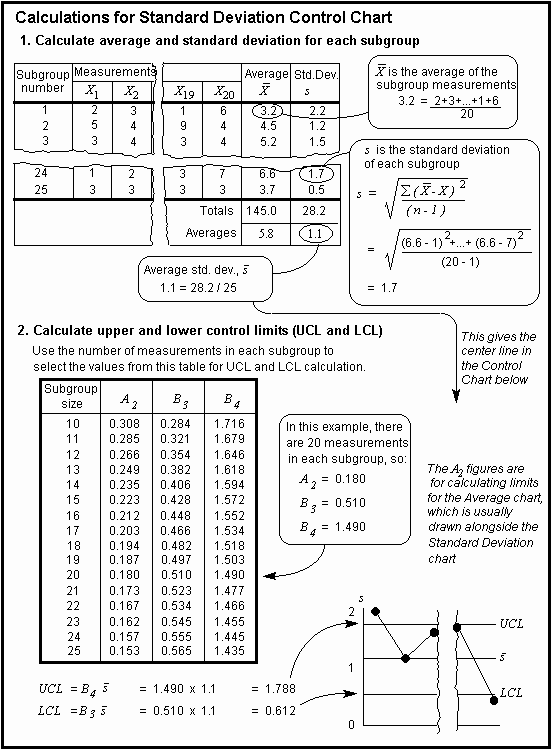The Psychology of Quality and More

# Calculation detail for X-bar/S Control Charts

Quality Tools > Tools of the Trade > Calculation detail for X-bar/S Control Charts

1. Calculate average and standard deviation for each subgroup in the standard manner (as a normal standard deviation calculation).

2. Calculate the upper and lower control limits. This again requires a magic constant to be used, based on the number of measures in each subgroup. A table for this is included in Fig. 3.
Note: the X-bar chart is calculated in exactly the same way as for the average and range charts.Calculating X-bar/S control limits

This article first appeared in Quality World, the journal of the Chartered Quality Institute

### You can buy books here

And the big
paperback book